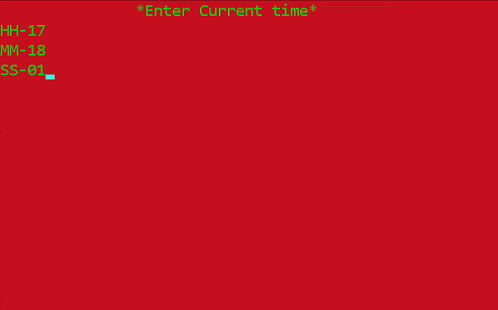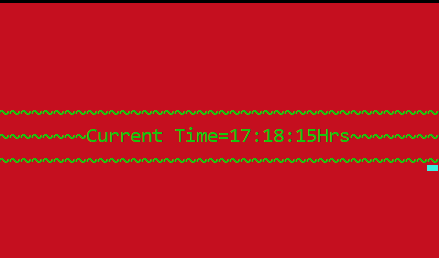# Digital Clock starting from user set time in C++

In this article, we will discuss the Digital Clock in C++ Language. It is an application that allows for a personal clock that starts at a custom set time and shows the time from that point onwards. This article describes how to make such a clock in a 24-hour format with HH:MM:SS slots and start the time from where one would want it to be, and then it moves forward from there.

Features: It is a Simple Digital clock developed using basic C++ concepts that shows hour, minute, and second.

Approach: The requirements for this program are just the basic concepts of the data types, variables, manipulators, Control statements, Conditional statements, etc. Below are the steps:

• Create a screen that will show “Current time” of your location which will be implemented using simple output methods used in C++ i.e., cout, and a manipulator “setw()“.
• In the fore mentioned screen, implement the HH column, MM column, SS column, that will contain the time.
• Implement colors using System(“color 4A”), the color will be in hexadecimal format and the console can be used to implement them using double-digit hex codes (0 to F) which will, in turn, change the text color in the console of the output.
• In the last screen, a digital clock can be seen finally implemented and running from the inputted time.

Functions Used:

• System(“cls”): It is used to clear the Console or the Screen. It can be avoided if anyone wants to see whatever is appearing on the screen.
• setw(): This function is used to leave the space of particular characters that you can write in the parenthesis. It is declared in <iomanip> header file. Here setw(70) is used.
• System(“color 4A”): This function is used to make background RED and text as LIGHT GREEN.
• Sleep(): sleep is a function that is declared in <windows.h> header file. It actually suspends the execution of the program temporarily for a period of time in milliseconds.

Below is the implementation of the above approach:

## C++

 `// C++ program to illustrate the digital ` `// clock starting from the entered time ` ` `  `#include ` `#include ` `#include ` `#include ` `using` `namespace` `std; ` ` `  `// Driver Code ` `int` `main() ` `{ ` `    ``system``(``"color 4A"``); ` ` `  `    ``// Background color and Foreground ` `    ``int` `hour, min, sec; ` `    ``cout << setw(70) ` `         ``<< ``"*Enter Current time*\n"``; ` ` `  `    ``// Use of manipulator for taking ` `    ``// input from the user ` `    ``cout << ``"HH- "``; ` `    ``cin >> hour; ` `    ``cout << ``"MM- "``; ` `    ``cin >> min; ` `    ``cout << ``"SS- "``; ` `    ``cin >> sec; ` ` `  `    ``// Background color and the ` `    ``// Foreground for 2nd screen ` `    ``system``(``"color 4A"``); ` ` `  `    ``// Cases for the Wrong Time Input ` `    ``if` `(hour > 23) { ` `        ``cout << ``"Wrong Time input"``; ` `    ``} ` `    ``else` `if` `(min > 60) { ` `        ``cout << ``"Wrong Time Input"``; ` `    ``} ` `    ``else` `if` `(sec > 60) { ` `        ``cout << ``"Wrong Time Input"``; ` `    ``} ` ` `  `    ``// Otherwise ` `    ``else` `{ ` `        ``while` `(1) ` ` `  `        ``// Run Block infinitely ` `        ``{ ` `            ``system``(``"cls"``); ` ` `  `            ``// Clear the console ` ` `  `            ``// Code for Showing Time ` `            ``for` `(hour; hour < 24; hour++) { ` ` `  `                ``for` `(min; min < 60; min++) { ` ` `  `                    ``for` `(sec; sec < 60; sec++) { ` `                        ``system``(``"cls"``); ` ` `  `                        ``cout << ``"\n\n\n\n~~~~~~~~~"` `                                ``"~~~~~~~~~~~~~~~~~~~~~"` `                                ``"~~~~~~~~~~~~~~~~~~"` `                                ``"Current Time = "` `                             ``<< hour << ``":"` `<< min << ``":"` `                             ``<< sec ` `                             ``<< ``"Hrs~~~~~~~~~~~~~~~~~~"` `                                ``"~~~~~~~~~~~~~~~~~~~~~"` `                                ``"~~~~~~~~~"``; ` ` `  `                        ``// HH:MM:SS columns in output ` ` `  `                        ``Sleep(1000); ` ` `  `                        ``// Pause for 1 sec ` `                    ``} ` `                    ``sec = 0; ` `                ``} ` `                ``min = 0; ` `            ``} ` `        ``} ` `    ``} ` `} `

Input:Output:Whether you're preparing for your first job interview or aiming to upskill in this ever-evolving tech landscape, GeeksforGeeks Courses are your key to success. We provide top-quality content at affordable prices, all geared towards accelerating your growth in a time-bound manner. Join the millions we've already empowered, and we're here to do the same for you. Don't miss out - check it out now!